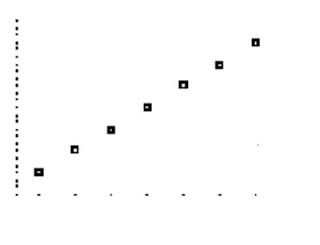## Wednesday, June 3, 2009

### June the Third Scribe

First of all we split into groups and Mr.K informed us that we were starting our new unit, sequences. We should be done this unit on Friday or Monday. So be prepared to move really fast!

Okay well apparently today was mostly a review from grade 10 (if you can remember that far back.. good job!)
On the first slide there were four sequences...

4, 7, 10, 13, __, __, __
3, 6, 12, 24, __, __, __
32, 16, 8, 4, __, __, __
1, 1, 2, 3, 5, 8, 13, __, __, __,

Mr. K wanted us to fill in the blanks and explain how we found the missing terms...

4, 7, 10, 13, 16, 19, 22 (Add 3 to the first term to find the second term. This is called an arithmetic sequence. )
3, 6, 12, 24, 48, 96, 192 (multiply the first term by 2 to find the second term. This is called a geometric sequence.)
32, 16, 8, 4, 2, 1, 1/2 (multiply by 1/2.)
1, 1, 2, 3, 5, 8, 13, 21, 34, 55 (this is the Fibonacci sequence! Add the first and second term together to find the third term.)

Now let's take a closer look and try to identify some patterns that can be applied to any other sequence we might encounter in the future.
4, 7, 10, 13, 16, 19, 22

Note: If you are asked to find the 37th term and you plan on adding 3's, you must add 36 threes.How do we get 3n + 1 ?
Well since we found the y-intercept we can graph this.By looking at this graph we can see that the slope is 3. So for future reference remember that our slope is the constant. So let's make an equation for this sequence.
tn= 3n+1
Where 3 is the slope and 1 is the y-intercept.

Everytime we have an arithmetic sequence it will be a linear function.This is the graph of our sequence. Here is how you would make that on your calculator! Hit stat, edit. Under L1 enter your rank (1-7) under L2 enter your values (4, 7, 10, 13, 16, 19, 22) Now hit 2nd stat plot (top left corner of the calculator). Hit enter and make sure plot 1 is on, under type make sure the dots are selected. Then hit graph.

Next we covered some definitions:
Recursive: Repeats again and again.
Implicit Definition: This is the teenage way of saying hi, it's an implied hello. Only clear to people who know what they're looking for.
The Common Difference (d): The number that is repeatedly added to successive terms in an arithmetic sequence.
Common Ratio: The number that isrepeatedly multiplied to successive terms in a geometric sequence.

How to find the nth term in an arithmetic sequence.
tn= a + (n-1)d
Where tn is the nth term
a is the first term
n is the rank of the nth term in the sequence
d is the common difference

How to find the nth term in an geometric sequence.
tn= ar^(n-1)
Where tn is the nth term
a is the first term
n is the rank of the nth term in the sequence
r is the common ratio

Here is the next sequence we looked at, 11, 5, -1, -7...
We were asked to find the 51 term.
Look at the difference between the terms.
5-11= -6
-1-5= -6
Therefore we know our constant is -6. Remember we're not subtracting 6 from the first term to get the second term, we are adding -6! :D

Okay so how to find the 51 term. First let's make a formula.

tn= a+ (n-1)-d
Where n is the term. a is the first value and d is the difference. So we have..

t51= 11+ (51-1)(-6)
= -289

Next we looked at the sequence 3, 6, 12, 24, 48, 96, 192
Notice the difference is not constant, so this is not an arithmetic sequence.

To make an equation for this kind of sequence we use the formula, tn= ar^(n-1)
a= the first term r= ratio
The ratio for this sequence is 2 because 6/3=2 and 12/6=2 etc.
So the implicit definition is tn= 3(2)^(n-1)

Next question! 32, 16, 8, 4, 2, 1, 1/2
We multiply by 1/2 to get the next term so 1/2 is our ratio. Now we find the 10 term.
tn= ar^(n-1)
t10= 31(1/2)^10-1)
t10= 1/16

Remember!!
If differences in sequences are different it is NOT arithmetic.
If there are common ratios it is geometric.

And the last slide..Um next scribe is Mary.

Homework is exercise 44 and 45.